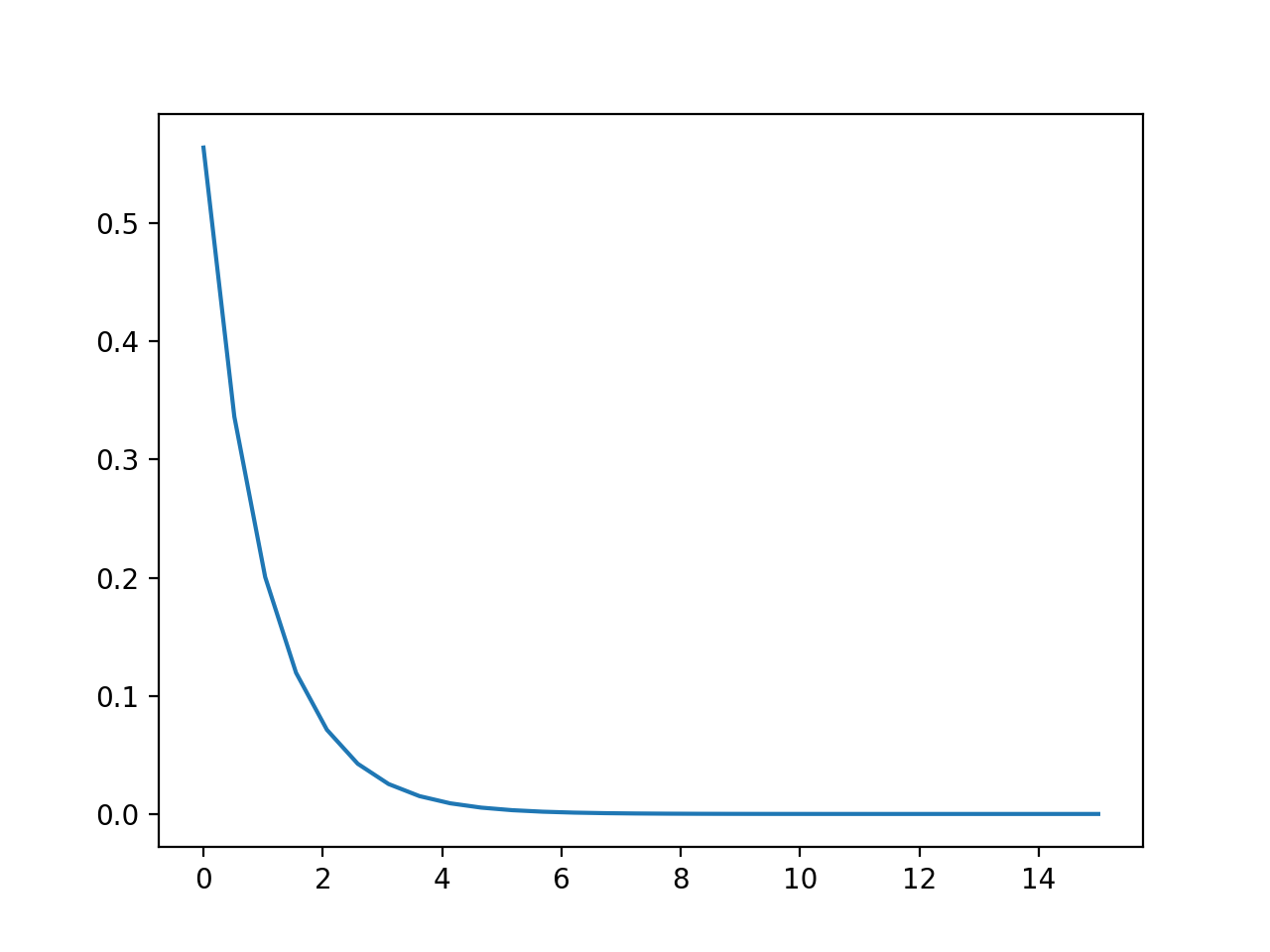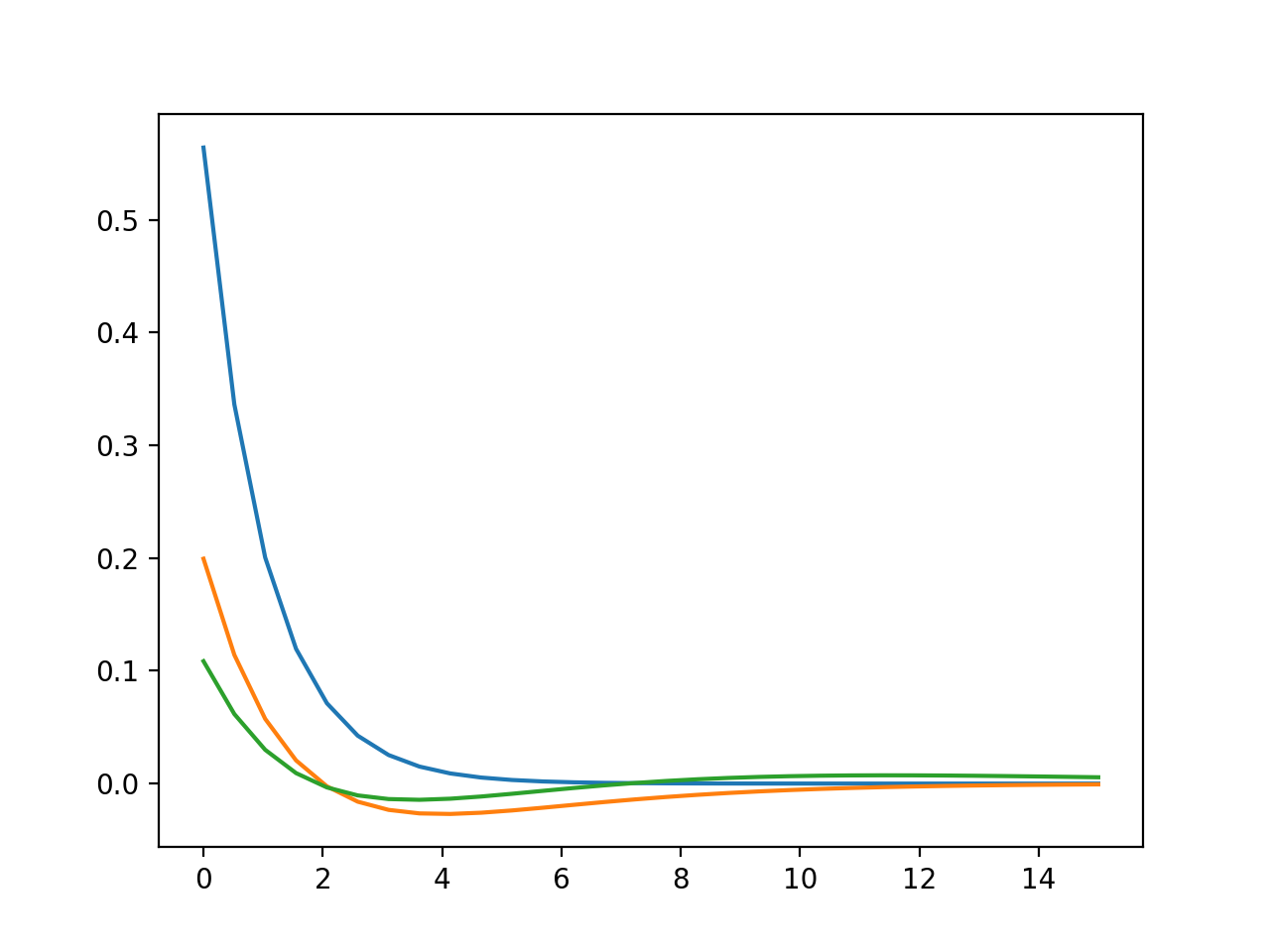# Plotting with Matplotlib’s Procedural Interface#

The first type of plot we will create using matplotlib are line and scatter plots. These are plots where we specify y values, or x and y values.

We will use the data file we discussed in the previous section to create the plots, s_orbitals_1D.csv. This data file contains the value of the 1s, 2s, and 3s orbital as a function of distance from the center of the atom (r).

We’ll start by importing the libraries we will need - pandas for reading the data file, and matplotlib for creating the plot. We will read in our data using the pd.read_csv function that we used in the previous lesson.

The first plots we create will use matplotlib’s procedural interface. This interface is designed to mimic MATLAB’s plotting procedure.

import pandas as pd

import matplotlib.pyplot as plt


s_orbitals.head()

r 1s 2s 3s
0 0.000000 0.564190 0.199471 0.108578
1 0.517241 0.336349 0.114183 0.061683
2 1.034483 0.200519 0.057408 0.029966
3 1.551724 0.119542 0.020580 0.009313
4 2.068966 0.071266 -0.002445 -0.003390

To make our plots interactive in the notebook, we use something called a jupyter notebook “magic” command. These commands start with a percent sign (%). These are not python commands, they are special for the jupyter notebook. The following will make our plots interactive. There is no output from this command.

%matplotlib notebook


When using the procedural interface, you always use commands which start with plt.function_name. To create a line plot, we use first create a figure using plt.figure, then we add data to the figure using plt.plot

plt.figure()
plt.plot(s_orbitals["r"], s_orbitals["1s"])[<matplotlib.lines.Line2D at 0x7fbd43636dc0>]


The line will be plotted on the most recently created figure.

If we want to add more data to oour figure, we use additional plt.plot commands.

plt.figure()
plt.plot(s_orbitals["r"], s_orbitals["1s"])
plt.plot(s_orbitals["r"], s_orbitals["2s"])
plt.plot(s_orbitals["r"], s_orbitals["3s"])[<matplotlib.lines.Line2D at 0x7fbd44c0a9d0>]

l